Home

# MORE ON A CERTAIN ARITHMETICAL DETERMINANT

## Abstract

Let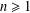$n\geq 1$ be an integer and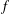$f$ be an arithmetical function. Let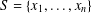$S=\{x_{1},\ldots ,x_{n}\}$ be a set of$n$ distinct positive integers with the property that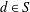$d\in S$ if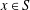$x\in S$ and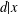$d|x$ . Then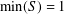$\min (S)=1$ . Let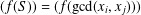$(f(S))=(f(\gcd (x_{i},x_{j})))$ and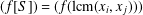$(f[S])=(f(\text{lcm}(x_{i},x_{j})))$ denote the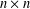$n\times n$ matrices whose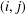$(i,j)$ -entries are$f$ evaluated at the greatest common divisor of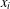$x_{i}$ and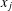$x_{j}$ and the least common multiple of$x_{i}$ and$x_{j}$ , respectively. In 1875, Smith [‘On the value of a certain arithmetical determinant’, Proc. Lond. Math. Soc. 7 (1875–76), 208–212] showed that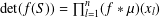$\det (f(S))=\prod _{l=1}^{n}(f\ast \unicode[STIX]{x1D707})(x_{l})$ , where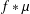$f\ast \unicode[STIX]{x1D707}$ is the Dirichlet convolution of$f$ and the Möbius function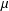$\unicode[STIX]{x1D707}$ . Bourque and Ligh [‘Matrices associated with classes of multiplicative functions’, Linear Algebra Appl. 216 (1995), 267–275] computed the determinant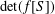$\det (f[S])$ if$f$ is multiplicative and, Hong, Hu and Lin [‘On a certain arithmetical determinant’, Acta Math. Hungar. 150 (2016), 372–382] gave formulae for the determinants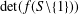$\det (f(S\setminus \{1\}))$ and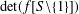$\det (f[S\setminus \{1\}])$ . In this paper, we evaluate the determinant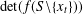$\det (f(S\setminus \{x_{t}\}))$ for any integer$t$ with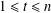$1\leq t\leq n$ and also the determinant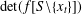$\det (f[S\setminus \{x_{t}\}])$ if$f$ is multiplicative.

## Footnotes

Hide All

The research was partially supported partially by National Science Foundation of China Grant Nos. 11371260, 11771304 and 11671218.

## References

Hide All
MathJax
MathJax is a JavaScript display engine for mathematics. For more information see http://www.mathjax.org.

# MORE ON A CERTAIN ARITHMETICAL DETERMINANT

## Metrics

### Full text viewsFull text views reflects the number of PDF downloads, PDFs sent to Google Drive, Dropbox and Kindle and HTML full text views.

Total number of HTML views: 0
Total number of PDF views: 0 *Loading metrics...

### Abstract viewsAbstract views reflect the number of visits to the article landing page.

Total abstract views: 0 *Loading metrics...

* Views captured on Cambridge Core between <date>. This data will be updated every 24 hours.

Usage data cannot currently be displayed